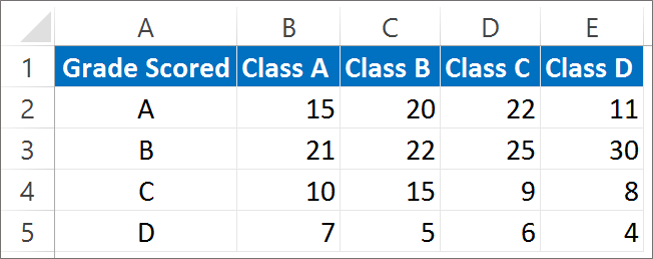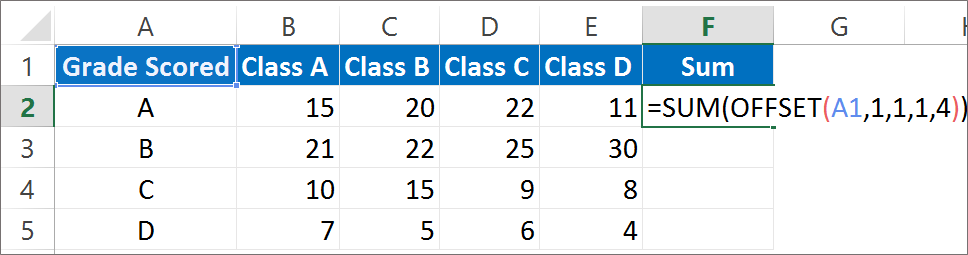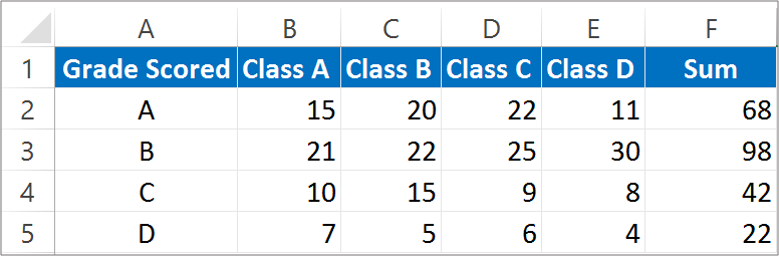# Offset function in Excel – Excel Formulas in Hindi

## Offset Function in Excel | How to use the Offset function?

The OFFSET function in Excel returns a reference to a range. It returns a range of cells that is specified number of rows and columns from the specified range. This function is very good for creating dynamic ranges and getting the range from the starting cell.

It is an in-built function in Excel and categorized under the head Lookup/References functions. It is entered as a part of the formula as it is a worksheet function. This function applies to all Excel version from the Excel 2000.

The Syntax for OFFSET function:

OFFSET(range, rows, columns, [height], [width])

Where,

Range: The starting point from where you will apply the OFFSET function.

Rows: The number of rows to be applied as the offset range. It can be positive or negative number.

Columns: The number of columns to be applied as the offset range. It can be positive or negative number.

Height: This is an optional argument. If you omit this argument it will take the height of the range into consideration this argument specifies the height of the returned range.

Width: This argument too is optional. If it is omitted it will assume the width of the range. This argument specifies the width of the returned range.

Note: If the returned range is beyond the valid cells the OFFSET function will return an error.

To get a basic idea about how the OFFSET function works, let’s take an example which will make your understanding more clear. Below is the example of the grade secured by students of 4 different class.Let us suppose that we want to find Offset by taking B3 as the reference. So the formula will be entered as =OFFSET (B3, 2, 2, 1, 1)

Where,

B3- stand for the reference range.

2- Stands for 2 rows down of B3.

2- Stands for 2 columns to the right of B3.

Here Height and width are taken as 1. It means 1 row x 1 column.

So the answer that we get is 6.

6 is the cell reference. Which is for the cell D5.

Note: No cells are moved when we get the answer, it is only the reference.

### Offset Function along with Sum function

The sum function along with the OFFSET function will calculate the Sum of the range provided for the OFFSET function. The syntax to calculate Sum for the Offset will be the same. Just enter the Sum formula ahead of the OFFSET function.

We want to calculate how many students scored grade A from all the 4 classes. Here we enter the formula for grade A as: =SUM(OFFSET(A1,1,1,1,4))Where,

A1: is the reference cell.

1 stands for 1 row below the reference range A1.

1 stand for 1 column to the right of the reference cell A1.

1 stand for the height of the row.

4 stand for the width.

It will calculate the sum of last n values.

The answer that we get is 68. We read it as there are a total of 68 students with Grade A taking sum of all the 4 divisions. We get the sum of (15 + 20+ 22 + 11)The Offset function can also be used along with Average, Min, Max. The OFFSET function creates a dynamic range and that range expands or contracts depending upon whether users adds or remove the data. Here you might have got a bit scenario of this function.

There are three alternatives to the OFFSET function. They are Excel tables, Excel Index Function, Excel Indirect Function.

Hope you like our tutorial for more cool and amazing trick of excel Like our Excel Superstar Facebook Page and subscribe to our Excel Superstar YouTube channel. Excel Superstar is the leading online training company, which provides Online Excel Course in Hindi. Connect with us and become an Excel Superstar.